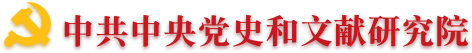•• 理論中國網   |
•"黨的文獻"公眾號"黃城根下"公眾號"黨的歷史"公眾號
•（微博）黨史網

“振興中華”這個口號，是孫中山在中日甲午戰爭期間第一次喊出來的。10年后，他發表了一篇向美國人民呼吁的文章——《中國問題的真解決》。在文中他動情地寫道：“一旦我們革新中國的偉大目標得以完成，不但在我們的美麗的國家將會出現新紀元的曙光，整個人類也將得以共享更為光明的前景”。這些話是他在100多年前中華民族正處在苦難深淵中時說的，但充滿著民族自豪和民族自信的激情，沒有半點半殖民地半封建國家常見的一味崇外媚外的可憐相。這正是堂堂正正的中國人最需要的風骨和品格，也是孫中山能吸引那麼多人追隨他共同奮斗的重要原因。

（作者為中央文獻研究室原常務副主任）

（來源：《 人民日報 》20161111日）

專家學者

• 翻譯
• C
• D
• F
• G
• H
• J
• L
• M
• O
• P
• Q
• S
• T
• W
• X
• Y
• Z
• C
• F
• G
• H
• J
• L
• P
• Q
• S
• T
• W
• X
• Y
• Z
• C
• D
• G
• H
• J
• L
• Q
• S
• W
• X
• Y
• Z